Open in App
Not now

# Introduction to Binary Tree – Data Structure and Algorithm Tutorials

• Difficulty Level : Easy
• Last Updated : 15 Mar, 2023

A tree is a popular data structure that is non-linear in nature. Unlike other data structures like an array, stack, queue, and linked list which are linear in nature, a tree represents a hierarchical structure. The ordering information of a tree is not important. A tree contains nodes and 2 pointers. These two pointers are the left child and the right child of the parent node. Let us understand the terms of tree in detail.

• Root: The root of a tree is the topmost node of the tree that has no parent node. There is only one root node in every tree.
• Parent Node:  The node which is a predecessor of a node is called the parent node of that node.
• Child Node: The node which is the immediate successor of a node is called the child node of that node.
• Sibling: Children of the same parent node are called siblings.
• Edge: Edge acts as a link between the parent node and the child node.
• Leaf: A node that has no child is known as the leaf node. It is the last node of the tree. There can be multiple leaf nodes in a tree.
• Subtree: The subtree of a node is the tree considering that particular node as the root node.
• Depth: The depth of the node is the distance from the root node to that particular node.
• Height: The height of the node is the distance from that node to the deepest node of that subtree.
• Height of tree: The Height of the tree is the maximum height of any node. This is the same as the height of the root node.
• Level: A level is the number of parent nodes corresponding to a given node of the tree.
• Degree of node:  The degree of a node is the number of its children.
• NULL: The number of NULL nodes in a binary tree is (N+1), where N is the number of nodes in a binary tree.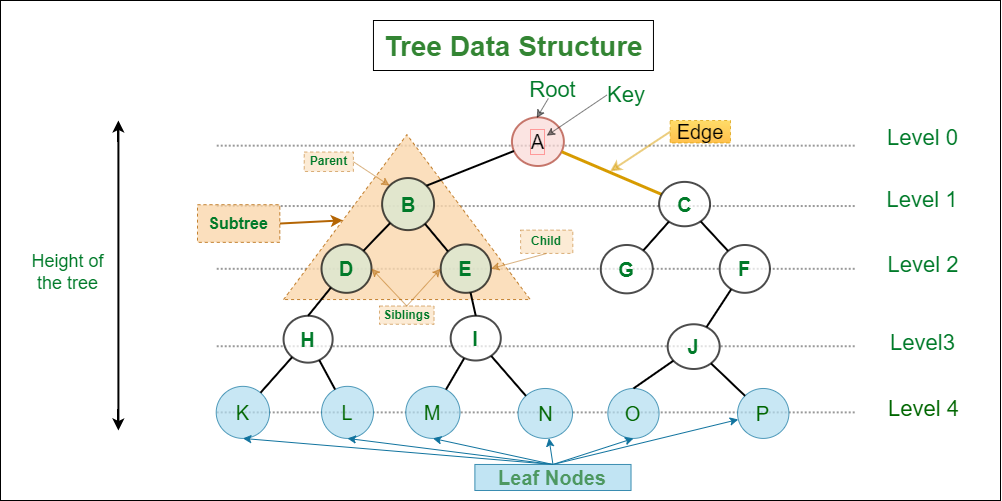Introduction to Binary Tree – Data Structure and Algorithm Tutorials

## Why to use Tree Data Structure?

1. One reason to use trees might be because you want to store information that naturally forms a hierarchy. For example, the file system on a computer: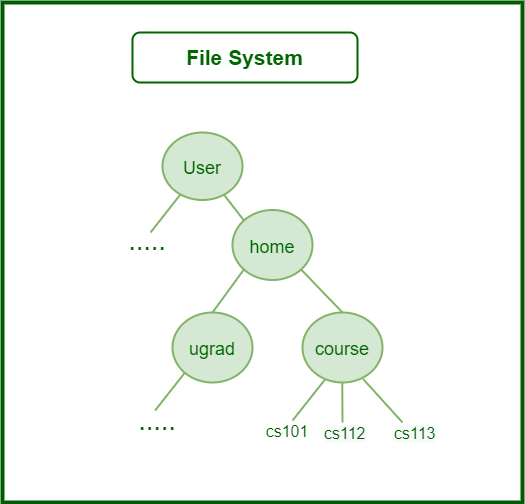File System

2. Trees (with some ordering e.g., BST) provide moderate access/search (quicker than Linked List and slower than arrays).
3. Trees provide moderate insertion/deletion (quicker than Arrays and slower than Unordered Linked Lists).
4. Like Linked Lists and unlike Arrays, Trees don’t have an upper limit on the number of nodes as nodes are linked using pointers.

## The main applications of tree data structure:

1. Manipulate hierarchical data.
2. Make information easy to search (see tree traversal).
3. Manipulate sorted lists of data.
4. As a workflow for compositing digital images for visual effects.
5. Router algorithms
6. Form of multi-stage decision-making (see business chess).
7. Trees can be used to represent the structure of a sentence, and can be used in parsing algorithms to analyze the grammar of a sentence.
8. Trees can be used to represent the decision-making process of computer-controlled characters in games, such as in decision trees.
9. Huffman coding uses a tree to represent the frequency of characters in a text, which can be used for data compression.
10. Trees are used to represent the syntax of a programming language, and can be used in compiler design to check the syntax of a program and generate machine code.

## What is a Binary Tree?

A binary tree is a tree data structure in which each node can have at most two children, which are referred to as the left child and the right child. The topmost node in a binary tree is called the root, and the bottom-most nodes are called leaves. A binary tree can be visualized as a hierarchical structure with the root at the top and the leaves at the bottom.

Binary trees have many applications in computer science, including data storage and retrieval, expression evaluation, network routing, and game AI. They can also be used to implement various algorithms such as searching, sorting, and graph algorithms.

## Representation of Binary Tree:

Each node in the tree contains the following:

• Data
• Pointer to the left child
• Pointer to the right child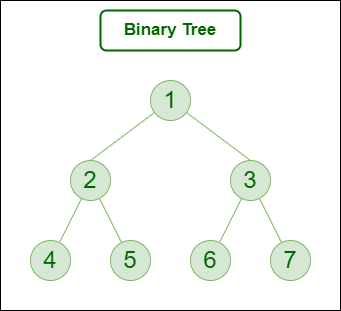Binary Tree

In C, we can represent a tree node using structures. In other languages, we can use classes as part of their OOP feature. Below is an example of a tree node with integer data.

## C

 `// Structure of each node of the tree ` ` `  `struct` `node { ` `    ``int` `data; ` `    ``struct` `node* left; ` `    ``struct` `node* right; ` `};`

## C++

 `// Use any below method to implement Nodes of tree ` ` `  `// Method 1: Using "struct" to make ` `// user-define data type ` `struct` `node { ` `    ``int` `data; ` `    ``struct` `node* left; ` `    ``struct` `node* right; ` `}; ` ` `  `// Method 2: Using "class" to make ` `// user-define data type ` `class` `Node { ` `public``: ` `    ``int` `data; ` `    ``Node* left; ` `    ``Node* right; ` `};`

## Python

 `# A Python class that represents  ` `# an individual node in a Binary Tree ` ` `  `class` `Node: ` `    ``def` `__init__(``self``, key): ` `        ``self``.left ``=` `None` `        ``self``.right ``=` `None` `        ``self``.val ``=` `key `

## Java

 `// Class containing left and right child ` `// of current node and key value ` `class` `Node { ` `    ``int` `key; ` `    ``Node left, right; ` ` `  `    ``public` `Node(``int` `item) ` `    ``{ ` `        ``key = item; ` `        ``left = right = ``null``; ` `    ``} ` `} `

## C#

 `// Class containing left and right child ` `// of current node and key value ` ` `  `class` `Node { ` `    ``int` `key; ` `    ``Node left, right; ` ` `  `    ``public` `Node(``int` `item) ` `    ``{ ` `        ``key = item; ` `        ``left = right = ``null``; ` `    ``} ` `} `

## Javascript

 ` `

## Basic Operations On Binary Tree:

• Inserting an element.
• Removing an element.
• Searching for an element.
• Deletion for an element.
• Traversing an element. There are four (mainly three) types of traversals in a binary tree which will be discussed ahead.

## Auxiliary Operations On Binary Tree:

• Finding the height of the tree
• Find the level of the tree
• Finding the size of the entire tree.

## Applications of Binary Tree:

• In compilers, Expression Trees are used which is an application of binary trees.
• Huffman coding trees are used in data compression algorithms.
• Priority Queue is another application of binary tree that is used for searching maximum or minimum in O(1) time complexity.
• Represent hierarchical data.
• Used in editing software like Microsoft Excel and spreadsheets.
• Useful for indexing segmented at the database is useful in storing cache in the system,
• Syntax trees are used for most famous compilers for programming like GCC, and AOCL to perform arithmetic operations.
• For implementing priority queues.
• Used to find elements in less time (binary search tree)
• Used to enable fast memory allocation in computers.
• Used to perform encoding and decoding operations.
• Binary trees can be used to organize and retrieve information from large datasets, such as in inverted index and k-d trees.
• Binary trees can be used to represent the decision-making process of computer-controlled characters in games, such as in decision trees.
•  Binary trees can be used to implement searching algorithms, such as in binary search trees which can be used to quickly find an element in a sorted list.
• Binary trees can be used to implement sorting algorithms, such as in heap sort which uses a binary heap to sort elements efficiently.

## Binary Tree Traversals:

Tree Traversal algorithms can be classified broadly into two categories:

• Depth-First Search (DFS) Algorithms

### Tree Traversal using Depth-First Search (DFS) algorithm can be further classified into three categories:

• Preorder Traversal (current-left-right): Visit the current node before visiting any nodes inside the left or right subtrees. Here, the traversal is root – left child – right child. It means that the root node is traversed first then its left child and finally the right child.
• Inorder Traversal (left-current-right): Visit the current node after visiting all nodes inside the left subtree but before visiting any node within the right subtree. Here, the traversal is left child – root – right child.  It means that the left child is traversed first then its root node and finally the right child.
• Postorder Traversal (left-right-current): Visit the current node after visiting all the nodes of the left and right subtrees.  Here, the traversal is left child – right child – root.  It means that the left child has traversed first then the right child and finally its root node.

### Tree Traversal using Breadth-First Search (BFS) algorithm can be further classified into one category:

• Level Order Traversal:  Visit nodes level-by-level and left-to-right fashion at the same level. Here, the traversal is level-wise. It means that the most left child has traversed first and then the other children of the same level from left to right have traversed.

Let us traverse the following tree with all four traversal methods: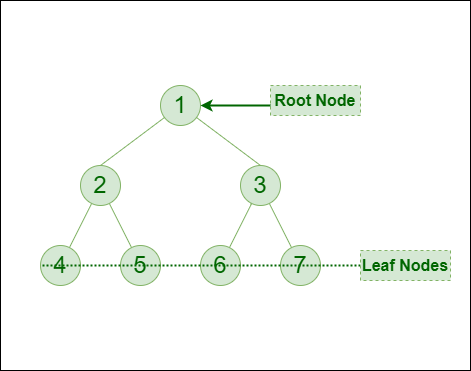Binary Tree

Pre-order Traversal of the above tree: 1-2-4-5-3-6-7
In-order Traversal of the above tree: 4-2-5-1-6-3-7
Post-order Traversal of the above tree: 4-5-2-6-7-3-1
Level-order Traversal of the above tree: 1-2-3-4-5-6-7

## Implementation of Binary Tree:

Let us create a simple tree with 4 nodes. The created tree would be as follows.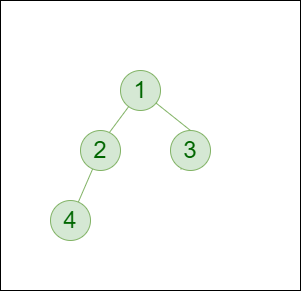Binary Tree

Simple example :

## C++

 `#include ` ` `  `struct` `Node { ` `  ``int` `data; ` `  ``Node* left; ` `  ``Node* right; ` ` `  `  ``Node(``int` `data) { ` `    ``this``->data = data; ` `    ``this``->left = nullptr; ` `    ``this``->right = nullptr; ` `  ``} ` `}; ` ` `  `class` `BinaryTree { ` ` ``public``: ` `  ``Node* root; ` ` `  `  ``BinaryTree() { ` `    ``root = nullptr; ` `  ``} ` ` `  `  ``void` `addNode(``int` `data) { ` `    ``Node* newNode = ``new` `Node(data); ` ` `  `    ``if` `(root == nullptr) { ` `      ``root = newNode; ` `    ``} ``else` `{ ` `      ``Node* focusNode = root; ` `      ``Node* parent; ` ` `  `      ``while` `(``true``) { ` `        ``parent = focusNode; ` ` `  `        ``if` `(data < focusNode->data) { ` `          ``focusNode = focusNode->left; ` `          ``if` `(focusNode == nullptr) { ` `            ``parent->left = newNode; ` `            ``return``; ` `          ``} ` `        ``} ``else` `{ ` `          ``focusNode = focusNode->right; ` `          ``if` `(focusNode == nullptr) { ` `            ``parent->right = newNode; ` `            ``return``; ` `          ``} ` `        ``} ` `      ``} ` `    ``} ` `  ``} ` ` `  `  ``void` `preOrderTraversal(Node* focusNode) { ` `    ``if` `(focusNode != nullptr) { ` `      ``std::cout << focusNode->data << ``" "``; ` `      ``preOrderTraversal(focusNode->left); ` `      ``preOrderTraversal(focusNode->right); ` `    ``} ` `  ``} ` `}; ` ` `  `int` `main() { ` `  ``BinaryTree tree; ` ` `  `  ``tree.addNode(50); ` `  ``tree.addNode(25); ` `  ``tree.addNode(75); ` `  ``tree.addNode(12); ` `  ``tree.addNode(37); ` `  ``tree.addNode(43); ` `  ``tree.addNode(30); ` ` `  `  ``tree.preOrderTraversal(tree.root); ` ` `  `  ``return` `0; ` `} `

## Java

 `class` `Node { ` `  ``int` `data; ` `  ``Node left; ` `  ``Node right; ` ` `  `  ``public` `Node(``int` `data) { ` `    ``this``.data = data; ` `  ``} ` `} ` ` `  `class` `BinaryTree { ` `  ``Node root; ` ` `  `  ``public` `void` `addNode(``int` `data) { ` `    ``Node newNode = ``new` `Node(data); ` ` `  `    ``if` `(root == ``null``) { ` `      ``root = newNode; ` `    ``} ``else` `{ ` `      ``Node focusNode = root; ` `      ``Node parent; ` ` `  `      ``while` `(``true``) { ` `        ``parent = focusNode; ` ` `  `        ``if` `(data < focusNode.data) { ` `          ``focusNode = focusNode.left; ` `          ``if` `(focusNode == ``null``) { ` `            ``parent.left = newNode; ` `            ``return``; ` `          ``} ` `        ``} ``else` `{ ` `          ``focusNode = focusNode.right; ` `          ``if` `(focusNode == ``null``) { ` `            ``parent.right = newNode; ` `            ``return``; ` `          ``} ` `        ``} ` `      ``} ` `    ``} ` `  ``} ` ` `  `  ``public` `void` `preOrderTraversal(Node focusNode) { ` `    ``if` `(focusNode != ``null``) { ` `      ``System.out.print(focusNode.data + ``" "``); ` `      ``preOrderTraversal(focusNode.left); ` `      ``preOrderTraversal(focusNode.right); ` `    ``} ` `  ``} ` `} ` ` `  `public` `class` `Main { ` `  ``public` `static` `void` `main(String[] args) { ` `    ``BinaryTree tree = ``new` `BinaryTree(); ` ` `  `    ``tree.addNode(``50``); ` `    ``tree.addNode(``25``); ` `    ``tree.addNode(``75``); ` `    ``tree.addNode(``12``); ` `    ``tree.addNode(``37``); ` `    ``tree.addNode(``43``); ` `    ``tree.addNode(``30``); ` ` `  `    ``tree.preOrderTraversal(tree.root); ` `  ``} ` `} `

## Python3

 `# code ` `# Definition for a binary tree node ` ` `  `class` `Node: ` ` `  `    ``def` `__init__(``self``, data): ` ` `  `        ``self``.data ``=` `data ` ` `  `        ``self``.left ``=` `None` ` `  `        ``self``.right ``=` `None` ` `  `class` `BinaryTree: ` ` `  `    ``def` `__init__(``self``): ` ` `  `        ``self``.root ``=` `None` ` `  `         `  ` `  `    ``def` `add_node(``self``, data): ` ` `  `        ``new_node ``=` `Node(data) ` ` `  `        ``# If root is None, assign the new node to the root ` ` `  `        ``if` `self``.root ``is` `None``: ` ` `  `            ``self``.root ``=` `new_node ` ` `  `        ``else``: ` ` `  `            ``focus_node ``=` `self``.root ` ` `  `            ``parent ``=` `None` ` `  `            ``while` `True``: ` ` `  `                ``parent ``=` `focus_node ` ` `  `                 `  ` `  `                ``# If data is less than focus_node, assign focus_node to the left child ` ` `  `                ``if` `data < focus_node.data: ` ` `  `                    ``focus_node ``=` `focus_node.left ` ` `  `                    ``# If there's no left child, assign the new node to the left child ` ` `  `                    ``if` `focus_node ``is` `None``: ` ` `  `                        ``parent.left ``=` `new_node ` ` `  `                        ``return` ` `  `                ``else``: ` ` `  `                    ``focus_node ``=` `focus_node.right ` ` `  `                    ``# If there's no right child, assign the new node to the right child ` ` `  `                    ``if` `focus_node ``is` `None``: ` ` `  `                        ``parent.right ``=` `new_node ` ` `  `                        ``return` ` `  `    ``def` `pre_order_traversal(``self``, focus_node): ` ` `  `        ``if` `focus_node ``is` `not` `None``: ` ` `  `            ``print``(focus_node.data, end``=``" "``) ` ` `  `            ``self``.pre_order_traversal(focus_node.left) ` ` `  `            ``self``.pre_order_traversal(focus_node.right) ` ` `  `# Example usage ` ` `  `tree ``=` `BinaryTree() ` ` `  `tree.add_node(``50``) ` ` `  `tree.add_node(``25``) ` ` `  `tree.add_node(``75``) ` ` `  `tree.add_node(``12``) ` ` `  `tree.add_node(``37``) ` ` `  `tree.add_node(``43``) ` ` `  `tree.add_node(``30``) ` ` `  `tree.pre_order_traversal(tree.root)`

## Javascript

 `//Javascript Code ` ` ``class Node { ` `        ``constructor(data) { ` `          ``this``.data = data; ` `          ``this``.left = ``null``; ` `          ``this``.right = ``null``; ` `        ``} ` `      ``} ` ` `  `      ``class BinaryTree { ` `        ``constructor() { ` `          ``this``.root = ``null``; ` `        ``} ` ` `  `        ``addNode(data) { ` `          ``let newNode = ``new` `Node(data); ` ` `  `          ``if` `(``this``.root == ``null``) { ` `            ``this``.root = newNode; ` `          ``} ``else` `{ ` `            ``let focusNode = ``this``.root; ` `            ``let parent; ` ` `  `            ``while` `(``true``) { ` `              ``parent = focusNode; ` ` `  `              ``if` `(data < focusNode.data) { ` `                ``focusNode = focusNode.left; ` `                ``if` `(focusNode == ``null``) { ` `                  ``parent.left = newNode; ` `                  ``return``; ` `                ``} ` `              ``} ``else` `{ ` `                ``focusNode = focusNode.right; ` `                ``if` `(focusNode == ``null``) { ` `                  ``parent.right = newNode; ` `                  ``return``; ` `                ``} ` `              ``} ` `            ``} ` `          ``} ` `        ``} ` ` `  `        ``preOrderTraversal(focusNode) { ` `          ``if` `(focusNode != ``null``) { ` `            ``console.log(focusNode.data); ` `            ``this``.preOrderTraversal(focusNode.left); ` `            ``this``.preOrderTraversal(focusNode.right); ` `          ``} ` `        ``} ` `      ``} ` ` `  `      ``let tree = ``new` `BinaryTree(); ` ` `  `      ``tree.addNode(50); ` `      ``tree.addNode(25); ` `      ``tree.addNode(75); ` `      ``tree.addNode(12); ` `      ``tree.addNode(37); ` `      ``tree.addNode(43); ` `      ``tree.addNode(30); ` ` `  `      ``tree.preOrderTraversal(tree.root);`

## C#

 `using` `System; ` ` `  `class` `Node { ` `    ``public` `int` `data; ` `    ``public` `Node left; ` `    ``public` `Node right; ` ` `  `    ``public` `Node(``int` `data) ` `    ``{ ` `        ``this``.data = data; ` `        ``this``.left = ``null``; ` `        ``this``.right = ``null``; ` `    ``} ` `} ` ` `  `class` `BinaryTree { ` `    ``public` `Node root; ` ` `  `    ``public` `BinaryTree() { root = ``null``; } ` ` `  `    ``public` `void` `AddNode(``int` `data) ` `    ``{ ` `        ``Node newNode = ``new` `Node(data); ` ` `  `        ``if` `(root == ``null``) { ` `            ``root = newNode; ` `        ``} ` `        ``else` `{ ` `            ``Node focusNode = root; ` `            ``Node parent; ` ` `  `            ``while` `(``true``) { ` `                ``parent = focusNode; ` ` `  `                ``if` `(data < focusNode.data) { ` `                    ``focusNode = focusNode.left; ` `                    ``if` `(focusNode == ``null``) { ` `                        ``parent.left = newNode; ` `                        ``return``; ` `                    ``} ` `                ``} ` `                ``else` `{ ` `                    ``focusNode = focusNode.right; ` `                    ``if` `(focusNode == ``null``) { ` `                        ``parent.right = newNode; ` `                        ``return``; ` `                    ``} ` `                ``} ` `            ``} ` `        ``} ` `    ``} ` ` `  `    ``public` `void` `PreOrderTraversal(Node focusNode) ` `    ``{ ` `        ``if` `(focusNode != ``null``) { ` `            ``Console.Write(focusNode.data + ``" "``); ` `            ``PreOrderTraversal(focusNode.left); ` `            ``PreOrderTraversal(focusNode.right); ` `        ``} ` `    ``} ` `} ` ` `  `class` `Program { ` `    ``static` `void` `Main(``string``[] args) ` `    ``{ ` `        ``BinaryTree tree = ``new` `BinaryTree(); ` ` `  `        ``tree.AddNode(50); ` `        ``tree.AddNode(25); ` `        ``tree.AddNode(75); ` `        ``tree.AddNode(12); ` `        ``tree.AddNode(37); ` `        ``tree.AddNode(43); ` `        ``tree.AddNode(30); ` ` `  `        ``tree.PreOrderTraversal(tree.root); ` `    ``} ` `}`

Output

`50 25 12 37 30 43 75 `

Time Complexity: O(h), h is height of tree.
Auxiliary Space: O(h), h is height of tree.

Below is the Implementation of the binary tree:

## C++

 `#include ` `using` `namespace` `std; ` ` `  `class` `Node { ` `public``: ` `    ``int` `data; ` `    ``Node* left; ` `    ``Node* right; ` `    ``// Val is the key or the value that ` `    ``// has to be added to the data part ` `    ``Node(``int` `val) ` `    ``{ ` `        ``data = val; ` `        ``// Left and right child for node ` `        ``// will be initialized to null ` `        ``left = NULL; ` `        ``right = NULL; ` `    ``} ` `}; ` ` `  `int` `main() ` `{ ` `    ``/*create root*/` `    ``Node* root = ``new` `Node(1); ` ` `  `    ``/* following is the tree after above statement ` `    ``1 ` `    ``/ \ ` `    ``NULL NULL ` `    ``*/` `    ``root ->    left = ``new` `Node(2); ` `    ``root ->    right = ``new` `Node(3); ` ` `  `    ``/* 2 and 3 become left and right children of 1 ` `       ``1 ` `      ``/ \ ` `     ``2   3 ` `    ``/ \ / \ ` `    ``NULL NULL NULL NULL ` `    ``*/` `    ``root -> left -> left = ``new` `Node(4); ` `    ``/* 4 becomes left child of 2 ` `         ``1 ` `       ``/   \ ` `      ``2     3 ` `     ``/ \    / \ ` `    ``4 NULL NULL NULL ` `    ``/   \ ` `    ``NULL NULL ` `    ``*/` `    ``return` `0; ` `}`

## C

 `#include ` `#include ` ` `  `struct` `node { ` `    ``int` `data; ` `    ``struct` `node* left; ` `    ``struct` `node* right; ` `}; ` ` `  `/* newNode() allocates a new node ` `with the given data and NULL left ` `and right pointers. */` ` `  `struct` `node* newNode(``int` `data) ` `{ ` `    ``// Allocate memory for new node ` `    ``struct` `node* node ` `        ``= (``struct` `node*)``malloc``(``sizeof``(``struct` `node)); ` ` `  `    ``// Assign data to this node ` `    ``node->data = data; ` ` `  `    ``// Initialize left and ` `    ``// right children as NULL ` `    ``node->left = NULL; ` `    ``node->right = NULL; ` `    ``return` `(node); ` `} ` ` `  `int` `main() ` `{ ` `    ``/*create root*/` `    ``struct` `node* root = newNode(1); ` ` `  `    ``/* following is the tree after above statement ` `        ``1 ` `       ``/  \ ` `     ``NULL NULL ` `    ``*/` `    ``root->left = newNode(2); ` `    ``root->right = newNode(3); ` ` `  `    ``/* 2 and 3 become left and right children of 1 ` `            ``1 ` `           ``/ \ ` `          ``2   3 ` `         ``/ \ / \ ` `    ``NULL NULL NULL NULL ` `    ``*/` ` `  `    ``root->left->left = newNode(4); ` ` `  `    ``/* 4 becomes left child of 2 ` `     ``1 ` `    ``/ \ ` `   ``2   3 ` `  ``/  \ / \ ` `4 NULL NULL NULL ` `/   \ ` `NULL NULL ` `*/` `    ``getchar``(); ` `    ``return` `0; ` `}`

## Java

 `// Class containing left and right child ` `// of current node and key value ` `class` `Node { ` ` `  `    ``int` `key; ` ` `  `    ``Node left, right; ` `   ``public` `Node(``int` `item) ` `    ``{ ` `        ``key = item; ` `        ``left = right = ``null``; ` `    ``} ` `} ` ` `  `// A Java program to introduce Binary Tree ` ` `  `class` `BinaryTree { ` `    ``// Root of Binary Tree ` `    ``Node root; ` `    ``// Constructors ` `    ``BinaryTree(``int` `key) { root = ``new` `Node(key); } ` `    ``BinaryTree() { root = ``null``; } ` `    ``public` `static` `void` `main(String[] args) ` `    ``{ ` `        ``BinaryTree tree = ``new` `BinaryTree(); ` `        ``// create root ` `        ``tree.root = ``new` `Node(``1``); ` `        ``/* following is the tree after above statement ` `           ``1 ` `          ``/ \ ` `        ``null null */` ` `  `        ``tree.root.left = ``new` `Node(``2``); ` `        ``tree.root.right = ``new` `Node(``3``); ` `        ``/* 2 and 3 become left and right children of 1 ` `          ``1 ` `         ``/ \ ` `        ``2   3 ` `       ``/ \ / \ ` `  ``null null null null */` `        ``tree.root.left.left = ``new` `Node(``4``); ` `        ``/* 4 becomes left child of 2 ` `        ``1 ` `       ``/ \ ` `      ``2   3 ` `     ``/ \ / \ ` `  ``4 null null null ` `    ``/ \ ` `  ``null null ` `        ``*/` `    ``} ` `}`

## Python

 `# Python program to introduce Binary Tree ` `# A class that represents an individual node ` `# in a Binary Tree ` ` `  ` `  `class` `Node: ` `    ``def` `__init__(``self``, key): ` `        ``self``.left ``=` `None` `          ``self``.right ``=` `None` `          ``self``.val ``=` `key ` ` `  `if` `__name__ ``=``=` `'__main__'``: ` `    ``# Create root ` `    ``root ``=` `Node(``1``) ` `    ``''' following is the tree after above statement ` `    ``1 ` `    ``/ \ ` `    ``None None'''` `root.left ``=` `Node(``2``) ` `root.right ``=` `Node(``3``) ` ` `  `''' 2 and 3 become left and right children of 1 ` `1 ` `/ \ ` `2 3 ` `/ \ / \ ` `None None None None'''` ` `  `root.left.left ``=` `Node(``4``) ` `'''4 becomes left child of 2 ` `1 ` `/ \ ` `2 3 ` `/ \ / \ ` `4 None None None ` `/ \ ` `None None'''`

## C#

 `// A C# program to introduce Binary Tree ` `using` `System; ` `// Class containing left and right child ` `// of current node and key value ` `public` `class` `Node { ` `    ``public` `int` `key; ` `    ``public` `Node left, right; ` `    ``public` `Node(``int` `item) ` `    ``{ ` `        ``key = item; ` `        ``left = right = ``null``; ` `    ``} ` `} ` ` `  `public` `class` `BinaryTree { ` ` `  `    ``// Root of Binary Tree ` `    ``Node root; ` `    ``// Constructors ` `    ``BinaryTree(``int` `key) { root = ``new` `Node(key); } ` ` `  `    ``BinaryTree() { root = ``null``; } ` ` `  `    ``// Driver Code ` `    ``public` `static` `void` `Main(String[] args) ` ` `  `    ``{ ` `        ``BinaryTree tree = ``new` `BinaryTree(); ` `        ``// Create root ` `        ``tree.root = ``new` `Node(1); ` `        ``/* Following is the tree after above statement ` `           ``1 ` `          ``/ \ ` `        ``null null */` `        ``tree.root.left = ``new` `Node(2); ` `        ``tree.root.right = ``new` `Node(3); ` `        ``/* 2 and 3 become left and right children of 1 ` `             ``1 ` `            ``/ \ ` `           ``2   3 ` `          ``/ \ / \ ` `    ``null null null null */` ` `  `        ``tree.root.left.left = ``new` `Node(4); ` `        ``/* 4 becomes left child of 2 ` `           ``1 ` `          ``/ \ ` `         ``2   3 ` `        ``/ \ / \ ` `     ``4 null null null ` `       ``/ \ ` `    ``null null ` `*/` `    ``} ` `} ` ` `  `// This code is contributed by PrinciRaj1992`

## Javascript

 ``

Summary: Tree is a hierarchical data structure. Main uses of trees include maintaining hierarchical data, providing moderate access and insert/delete operations. Binary trees are special cases of tree where every node has at most two children.

Below are set 2 and set 3 of this post.
Properties of Binary Tree
Types of Binary Tree